Now you don't have to remember any of your passwords, and they are still safe and ready to use!

BeginnerShowcase (no instructions)25,458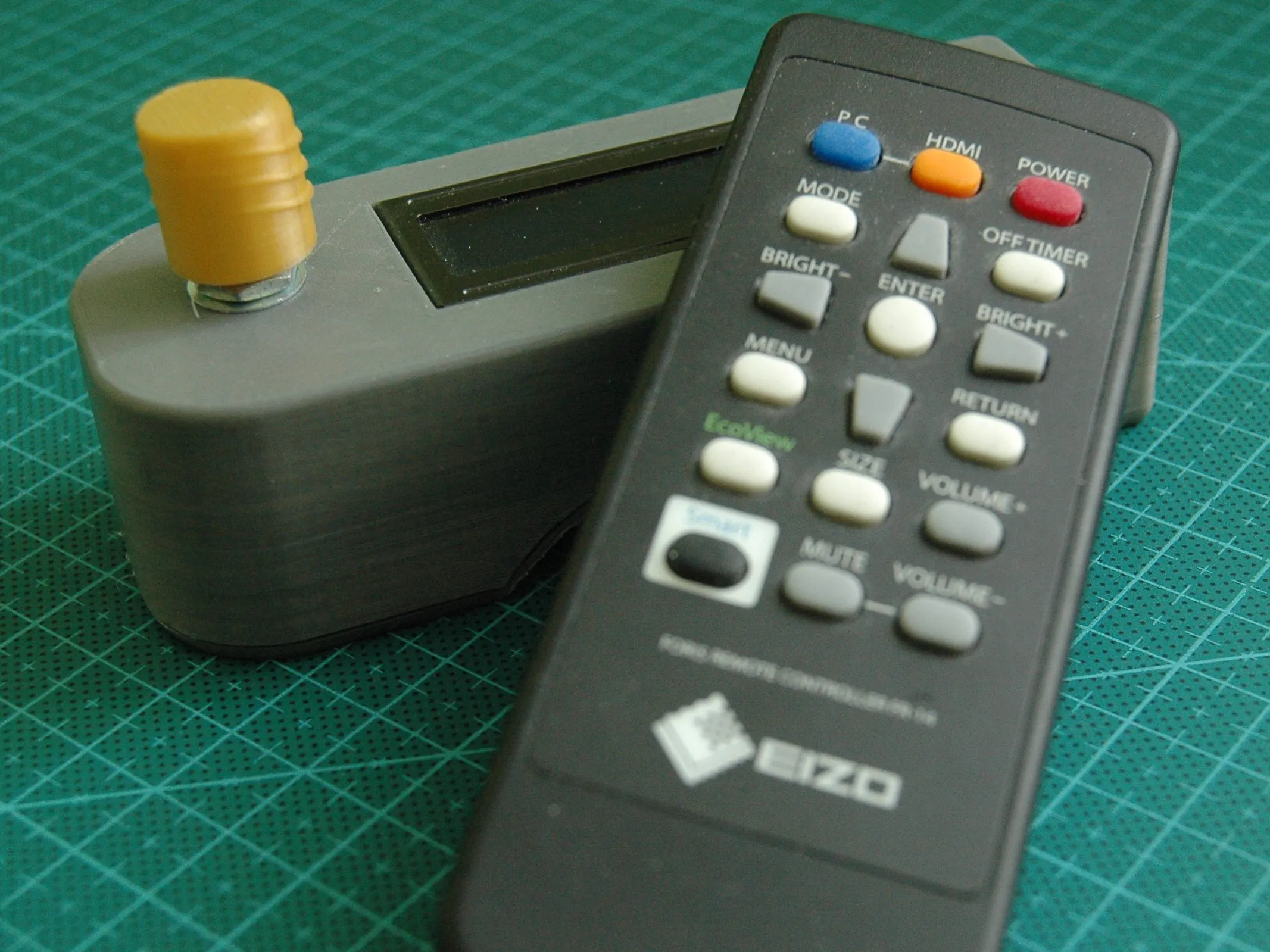## Things used in this project

### Hardware components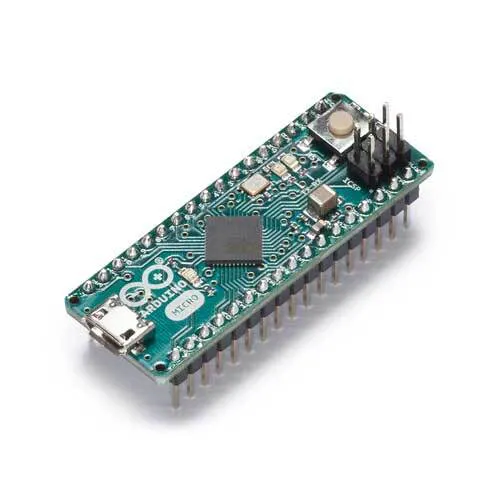Arduino Micro
×1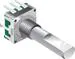Rotary Encoder with Push-Button
×1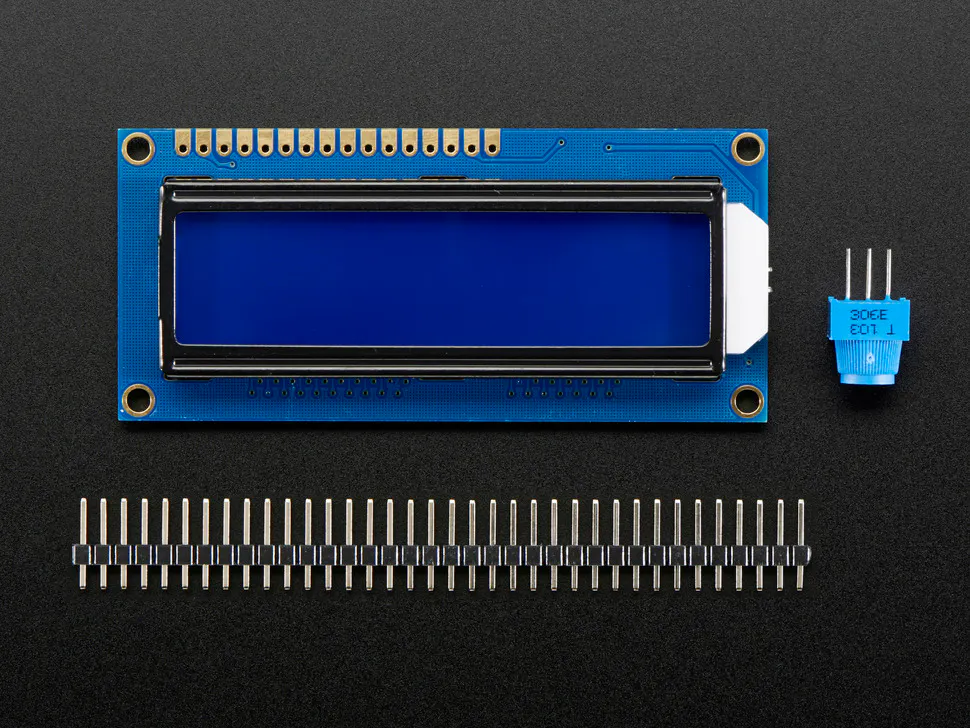Adafruit Standard LCD - 16x2 White on Blue
×1

### Software apps and online servicesArduino IDE

### Hand tools and fabrication machines3D Printer (generic)

## Schematics

### Schematic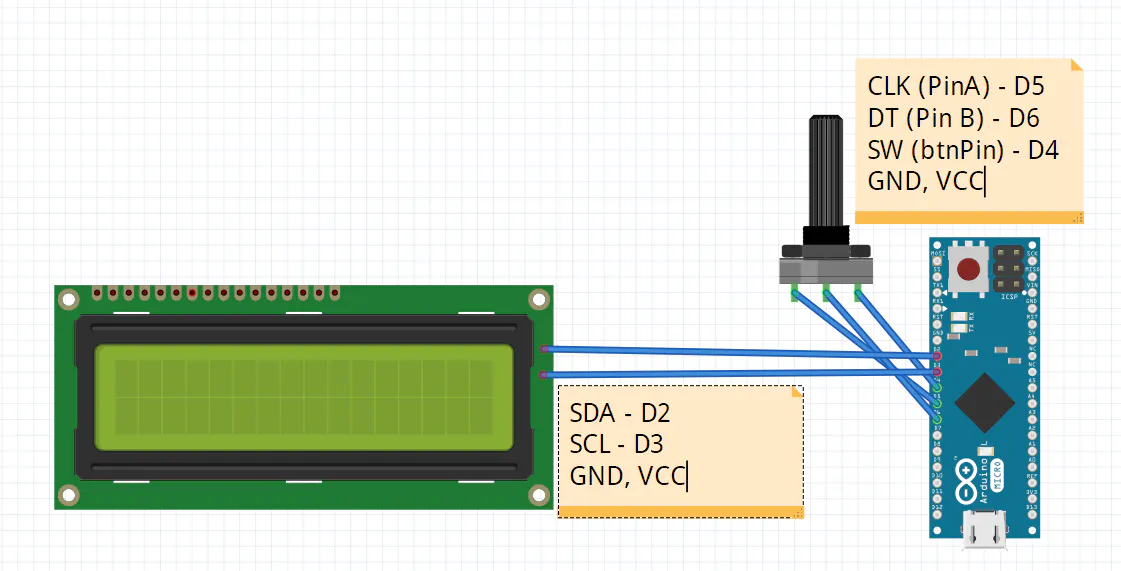## Code

### The code

C/C++
```#include <Keyboard.h>                                 //
#include <LiquidCrystal_I2C.h>                        //
#include <EEPROM.h>
#define pinA 5                                        //
#define pinB 6                                        //
#define btnPin 4                                      //

byte aState;                                          //
byte bState;                                          //
byte aLastState;                                      //
int dir;                                              //-1 -  . ., 1 -  . .
byte attempt = 0;                                     //-
int pos = 0;                                          //
byte typed = 0;                                       //
bool accepted = false;                                //

String code = "101";                                  //  : 101 -- 110011
int codeLen = 0;                                      //
String enter = "";                                    //

int a = 0;                                                //
int len = 0;                                              //
int counter;

long currentTime;

{"EPN", ""},
{"Mail", ""},
{"Asus", ";"},
{"GMX", ""}
};

LiquidCrystal_I2C lcd(0x3B, 16, 2);                   //

int lcdClear(byte x) {                                //
if (x == 2) {                                       //2 clear all, 0 clear first line, 1 clear second line
for (int i = 0; i < 2; ++i) {
lcd.setCursor(0, i);
lcd.print("                ");
}
}
else {
lcd.setCursor(0, x);
lcd.print("                ");
}
}

void setup() {
pinMode(pinA, INPUT);                               //  -
pinMode(pinB, INPUT);                               //  -
pinMode(btnPin, INPUT);                             // -
Serial.begin(9600);                                 //
Keyboard.begin();                                   //
lcd.init();                                         //
lcd.backlight();                                    //
lcd.setCursor(0, 0);
lcd.setCursor(1, 1);
lcd.print("Version 1.6 PM");
delay(3000);
lcdClear(2);
a++;
len++;
}
//Serial.println(len);
}

void getDirection() {
if (aState != aLastState) {                         // ...
if (aState == digitalRead(pinB)) {                           //  ...
//Serial.println("CCW 0");                        //
dir = -1;                                       //  . .
}
else {
//Serial.println("CW 1");                         //
dir = 1;                                        //  . .
}
}
aLastState = aState;                                //  .
}

void convert() {                                      //   ,
for (int k = 0; k < codeLen; k++) {
enter.remove(k, 1);
}
}

void check() {
if (enter == code) {
accepted = true;                                //
lcdClear(2);
lcd.setCursor(4, 0);
lcd.print("Welcome!");
delay(2000);
lcdClear(2);
enter = "";
typed = 0;
lcd.setCursor(0, 0);
lcd.print(">");
if (len > 1) {
lcd.setCursor(1, 1);
}
}
else {
attempt += 1;                                    //-
if (attempt == 3) {
lcdClear(2);
lcd.setCursor(0, 0);
lcd.setCursor(0, 1);
lcd.print("Wait 1 minute!");
currentTime = millis();
while (millis() - currentTime < 1000 * 60) {
currentTime = millis();
}
attempt = 0;
lcdClear(2);
typed = 0;
}
else {
lcd.setCursor(7, 0);
lcd.print("         ");
lcd.setCursor(15, 1);
lcd.print(3 - attempt);
lcd.setCursor(7, 0);
}
accepted = false;                                //
enter = "";
}
}

void loop() {
if (accepted == false) {
lcdClear(2);
lcd.setCursor(0,0);
lcd.print("      WAIT      ");
delay(1000 * 30);
lcdClear(2);
delay(1000);
}
getDirection();                                 //
if (typed == 0) {
lcd.setCursor(0, 0);
lcd.print("Unlock:");
lcd.setCursor(0, 1);
lcd.print("Attempts left: 3");
typed = 1;
lcd.setCursor(7, 0);
}
if (dir != 0) {
if (dir == -1) {
enter = enter + "0";
}
else {
enter = enter + "1";
}
codeLen = enter.length();                         //
if (codeLen % 2 == 0) {
lcd.print("*");
}
dir = 0;
}
if (digitalRead(btnPin) == 0) {                       //  ...
delay(300);
convert();
check();
}
}

else {
getDirection();
if (dir == 1 || dir == -1) {
counter++;
if (counter % 2 == 0) {
counter = 0;
if (dir == 1) {
if (pos + 1 < len) {
if (pos % 2 == 0) {
pos++;
lcd.setCursor(0, 0);
lcd.print(" ");
lcd.setCursor(0, 1);
lcd.print(">");
}
else {
pos++;
lcdClear(2);
lcd.setCursor(0, 0);
lcd.print(">");
if (pos + 1 <= len) {
lcd.setCursor(1, 1);
}
}
}
}
else {
if (pos - 1 >= 0) {
if (pos % 2 == 0) {
pos--;
lcdClear(2);
lcd.setCursor(0, 1);
lcd.print(">");
lcd.setCursor(1, 0);
}
else {
pos--;
lcd.setCursor(0, 1);
lcd.print(" ");
lcd.setCursor(0, 0);
lcd.print(">");
}
}
}
}
dir = 0;
}
delay(300);